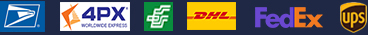•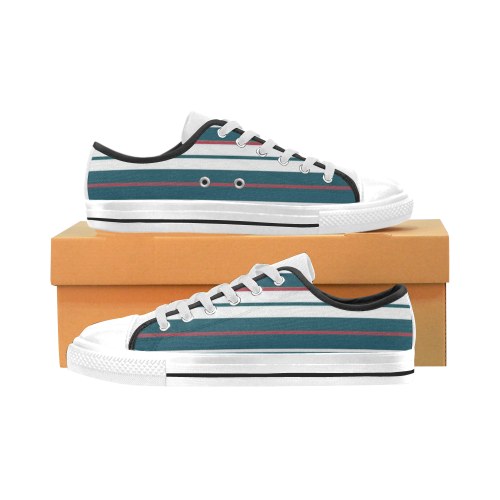•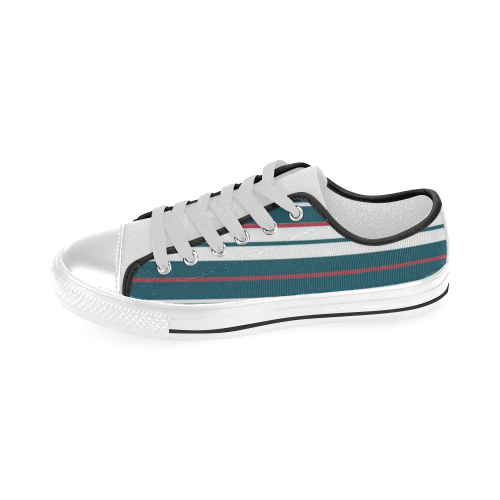•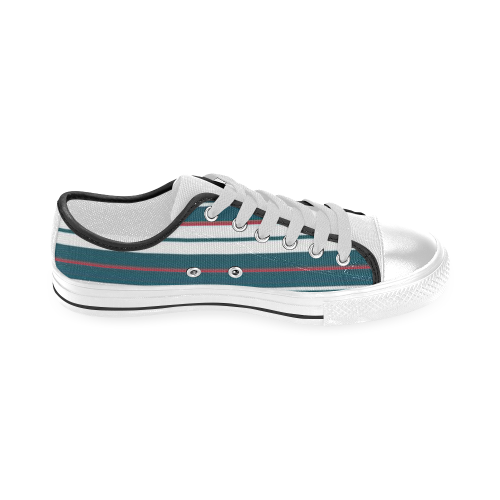•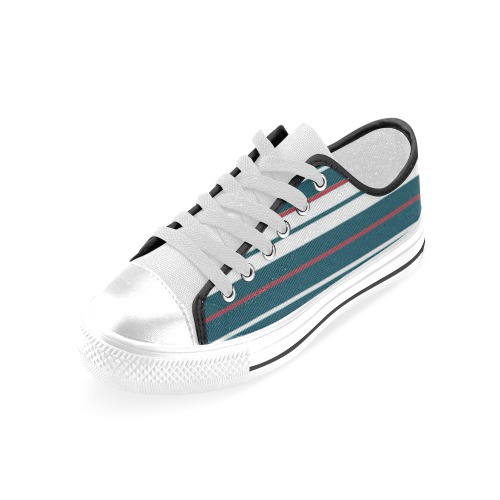### Aquila Canvas Men's Shoes (Model 018)

\$23.00 ~ \$23.00
Retail Price \$57.99 ~ \$57.99

Partial Shipping Included

•   White
•   Black

US4.5=EUR37=UK4=243mm=9.6inch
US5.5=EUR38=UK5=248mm=9.8inch
US6=EUR39=UK5.5 =252mm=9.9inch
US6.5=EUR39.5=UK6=256mm=10.1inch
US7.5=EUR40.5=UK7=265mm=10.4inch

US8=EUR41=UK7.5 =269mm=10.6inch

US8.5=EUR42=UK8 =273mm=10.8inch
US9=EUR42.5=UK8.5 =277mm=10.9inch
US9.5=EUR43=UK9 =281mm=11.1inch
US10=EUR44=UK9.5 =286mm=11.3inch
US10.5=EUR44.5=UK10 =290mm=11.4inch
US11=EUR45=UK11 =294mm=11.6inch
US12=EUR46=UK12 =303mm=11.9inch
US13=EUR48=UK13 =311mm=12.2inch
US14=EUR49=UK14 =315mm=12.4inch

Payment methods we accept: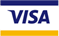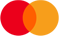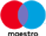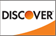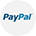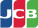We deliver with: